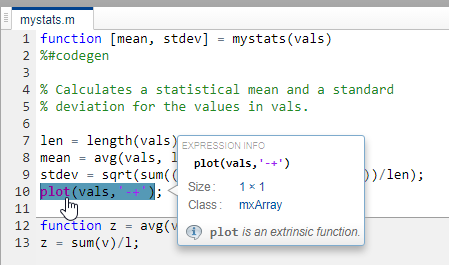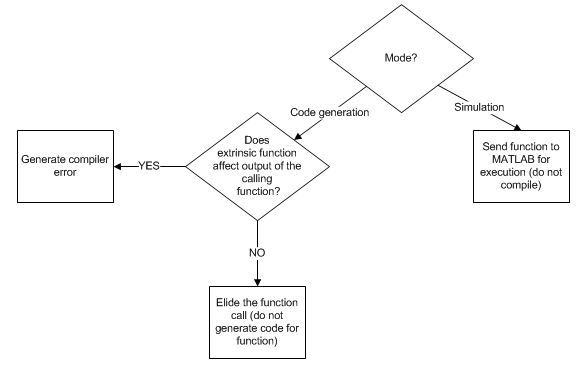## Extrinsic Functions

When processing a call to a function `foo` in your MATLAB® code, the code generator finds the definition of `foo` and generates code for its body. In some cases, you might want to bypass code generation and instead use the MATLAB engine to execute the call. Use `coder.extrinsic('foo')` to declare that calls to `foo` do not generate code and instead use the MATLAB engine for execution. In this context, `foo` is referred to as an extrinsic function. This functionality is available only when the MATLAB engine is available in MEX functions or during `coder.const` calls at compile time.

If you generate standalone code for a function that calls `foo` and includes `coder.extrinsic('foo')`, the code generator attempts to determine whether `foo` affects the output. If `foo` does not affect the output, the code generator proceeds with code generation, but excludes `foo` from the generated code. Otherwise, the code generator produces a compilation error.

The code generator automatically treats many common MATLAB visualization functions, such as `plot`, `disp`, and `figure`, as extrinsic. You do not have to explicitly declare them as extrinsic functions by using `coder.extrinsic`. For example, you might want to call `plot` to visualize your results in the MATLAB environment. If you generate a MEX function from a function that calls `plot`, and then run the generated MEX function, the code generator dispatches calls to the `plot` function to the MATLAB engine. If you generate a library or executable, the generated code does not contain calls to the `plot` function. The code generation report highlights calls from your MATLAB code to extrinsic functions so that it is easy to determine which functions are supported only in the MATLAB environment.For unsupported functions other than common visualization functions, you must declare the functions to be extrinsic (see Resolution of Function Calls for Code Generation). Extrinsic functions are not compiled, but instead executed in MATLAB during simulation (see Resolution of Extrinsic Functions During Simulation).

There are two ways to declare a function to be extrinsic:

### Declaring MATLAB Functions as Extrinsic Functions

To declare a MATLAB function to be extrinsic, add the `coder.extrinsic` construct at the top of the main function or a local function:

`coder.extrinsic('function_name_1', ... , 'function_name_n');`

#### Declaring Extrinsic Functions

The following code declares the MATLAB `patch` function extrinsic in the local function `create_plot`. You do not have to declare `axis` as extrinsic because `axis` is one of the common visualization functions that the code generator automatically treats as extrinsic.

```function c = pythagoras(a,b,color) %#codegen % Calculates the hypotenuse of a right triangle % and displays the triangle. c = sqrt(a^2 + b^2); create_plot(a, b, color); function create_plot(a, b, color) %Declare patch as extrinsic coder.extrinsic('patch'); x = [0;a;a]; y = [0;0;b]; patch(x, y, color); axis('equal');```

The code generator does not produce code for `patch` and `axis`, but instead dispatches them to MATLAB for execution.

To test the function, follow these steps:

1. Convert `pythagoras` to a MEX function by executing this command at the MATLAB prompt:

`codegen -report pythagoras -args {1, 1, [.3 .3 .3]}`

2. Click the link to the code generation report and then, in the report, view the MATLAB code for `create_plot`.

The report highlights the `patch` and `axis` functions to indicate that they are supported only within the MATLAB environment.3. Run the MEX function by executing this command:

`pythagoras_mex(3, 4, [1.0 0.0 0.0]);`

MATLAB displays a plot of the right triangle as a red patch object:#### When to Use the coder.extrinsic Construct

Use the `coder.extrinsic` construct to:

#### Rules for Extrinsic Function Declarations

Observe the following rules when declaring functions extrinsic for code generation:

• Declare the function extrinsic before you call it.

• Do not use the extrinsic declaration in conditional statements.

#### Scope of Extrinsic Function Declarations

The `coder.extrinsic` construct has function scope. For example, consider the following code:

```function y = foo %#codegen coder.extrinsic('rat','min'); [N D] = rat(pi); y = 0; y = min(N, D); ```

In this example, `rat` and `min` as treated as extrinsic every time they are called in the main function `foo`. There are two ways to narrow the scope of an extrinsic declaration inside the main function:

• Declare the MATLAB function extrinsic in a local function, as in this example:

```function y = foo %#codegen coder.extrinsic('rat'); [N D] = rat(pi); y = 0; y = mymin(N, D); function y = mymin(a,b) coder.extrinsic('min'); y = min(a,b); ```

Here, the function `rat` is extrinsic every time it is called inside the main function `foo`, but the function `min` is extrinsic only when called inside the local function `mymin`.

• Call the MATLAB function using `feval`, as described in Calling MATLAB Functions Using feval.

### Calling MATLAB Functions Using feval

The function `feval` is automatically interpreted as an extrinsic function during code generation. Therefore, you can use `feval` to conveniently call functions that you want to execute in the MATLAB environment, rather than compiled to generated code.

Consider the following example:

```function y = foo coder.extrinsic('rat'); [N D] = rat(pi); y = 0; y = feval('min', N, D); ```

Because `feval` is extrinsic, the statement ```feval('min', N, D)``` is evaluated by MATLAB — not compiled — which has the same result as declaring the function `min` extrinsic for just this one call. By contrast, the function `rat` is extrinsic throughout the function `foo`.

The code generator does not support the use of `feval` to call local functions or functions that are located in a private folder.

### Extrinsic Declaration for Nonstatic Methods

Suppose that you define a class `myClass` that has a nonstatic method `foo`, and then create an instance `obj` of this class. If you want to declare the method `obj.foo` as extrinsic in your MATLAB code that you intend for code generation, follow these rules:

• Write the call to `foo` as a function call. Do not write the call by using the dot notation.

• Declare `foo` to be extrinsic by using the syntax `coder.extrinsic('foo')`.

For example, define `myClass` as:

```classdef myClass properties prop = 1 end methods function y = foo(obj,x) y = obj.prop + x; end end end ```

Here is an example MATLAB function that declares `foo` as extrinsic.

```function y = myFunction(x) %#codegen coder.extrinsic('foo'); obj = myClass; y = foo(obj,x); end ```

Nonstatic methods are also known as ordinary methods. See Define Class Methods and Functions.

### Resolution of Extrinsic Functions During Simulation

The code generator resolves calls to extrinsic functions — functions that do not support code generation — as follows:During simulation, the code generator produces code for the call to an extrinsic function, but does not generate the internal code for the function. Therefore, you can run the simulation only on platforms where you install MATLAB software.

During code generation, the code generator attempts to determine whether the extrinsic function affects the output of the function in which it is called — for example by returning `mxArrays` to an output variable (see Working with mxArrays). Provided that the output does not change, code generation proceeds, but the extrinsic function is excluded from the generated code. Otherwise, the code generator issues a compiler error.

### Working with mxArrays

The output of an extrinsic function is an `mxArray` — also called a MATLAB array. The only valid operations for `mxArrays` are:

• Storing `mxArrays` in variables

• Passing `mxArrays` to functions and returning them from functions

• Converting `mxArrays` to known types at run time

To use `mxArrays` returned by extrinsic functions in other operations, you must first convert them to known types, as described in Converting mxArrays to Known Types.

#### Converting mxArrays to Known Types

To convert an `mxArray` to a known type, assign the `mxArray` to a variable whose type is defined. At run time, the `mxArray` is converted to the type of the variable assigned to it. However, if the data in the `mxArray` is not consistent with the type of the variable, you get a run-time error.

For example, consider this code:

```function y = foo %#codegen coder.extrinsic('rat'); [N D] = rat(pi); y = min(N, D); ```

Here, the top-level function `foo` calls the extrinsic function `rat`, which returns two `mxArrays` representing the numerator `N` and denominator `D` of the rational fraction approximation of `pi`. Although you can pass these `mxArrays` to another MATLAB function — in this case, `min` — you cannot assign the `mxArray` returned by `min` to the output `y`.

If you run this function `foo` in a MATLAB Function block in a Simulink® model, the code generates the following error during simulation:

`Function output 'y' cannot be of MATLAB type.`

To fix this problem, define `y` to be the type and size of the value that you expect `min` to return — in this case, a scalar double — as follows:

```function y = foo %#codegen coder.extrinsic('rat'); [N D] = rat(pi); y = 0; % Define y as a scalar of type double y = min(N,D);```

### Restrictions on Extrinsic Functions for Code Generation

The full MATLAB run-time environment is not supported during code generation. Therefore, the following restrictions apply when calling MATLAB functions extrinsically:

• MATLAB functions that inspect the caller, or read or write to the caller workspace do not work during code generation. Such functions include:

• The MATLAB debugger cannot inspect variables defined in extrinsic functions.

• Functions in generated code can produce unpredictable results if your extrinsic function performs the following actions at run time:

• Change folders

• Change the MATLAB path

• Delete or add MATLAB files

• Change warning states

• Change MATLAB preferences

• The code generator does not support the use of `coder.extrinsic` to call functions that are located in a private folder.

• The code generator does not support the use of `coder.extrinsic` to call local functions.

### Limit on Function Arguments

You can call functions with up to 64 inputs and 64 outputs.

## SupportGet trial now